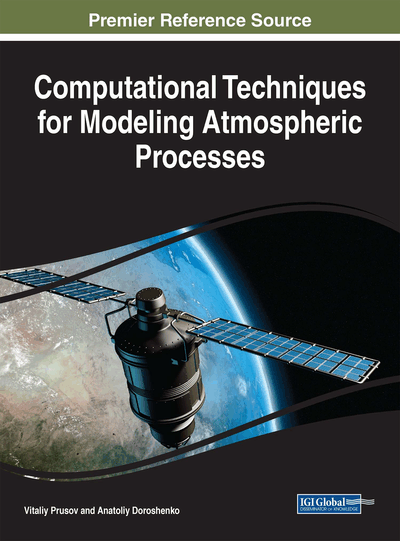# Equations of Hydrodynamics, Heat, and Mass Transfer in Models of Regional and Mesoscale Atmospheric Processes

DOI: 10.4018/978-1-5225-2636-0.ch005
Available
\$37.50
No Current Special Offers

## Abstract

The formation of meteorological processes and phenomena in the atmosphere in each specific area is influenced by the processes of different scales. However, the significance of each process in different cases is different. Therefore, there are certain priorities for the inclusion of certain processes in the hydrodynamic model. Depending on the size of the territory taken for modeling of processes or individual phenomena in the atmosphere, hydrodynamic models are divided into local and regional. In this chapter, we consider these features in terms of mathematical models.
Chapter Preview
Top

## Mesoscale And Regional Atmospheric Models

For mesoscale processes described by local and regional models, the basic equations of hydrodynamics are formulated in Cartesian and spherical coordinates, respectively. The vertical coordinate takes into the account the relief of the underlying surface.

Equations (3.2.10) have a number of specific features, which are exercised in the numerical implementation. One of the essential features of a space-elliptical nature of equations is due to the influence of the strain tensor divergencein the flow field. In this regard, for the solution of equations (3.2.10), typical methods for solving initial-boundary problems and the formulation of conditions on all the boundaries of the computational domain must be used.

The system of equations (3.2.10) at the termhas a parameter v, changing the value of which corresponds to a significant change in the smoothness of the solution due to the large vertical gradients of the meteorological variables at the underlying surface in the boundary layer.

Finally, the system of equations (3.2.10) is nonlinear. This non-linearity, typical of many systems of hydrodynamic type, is caused by the inertia components in the equations of momentum. In conjunction with the two features mentioned above, the nonlinear equations (3.2.10) leads to the formation of highly complex spatial-temporal structures. The numerical solution, in this case, is not only irregular in time, but it becomes much more complicated its spatial structure, in particular, it becomes unstable and breaks up the boundary layer, the secondary flows appear in the external flow (in the free atmosphere), etc. In some cases, it is difficult to distinguish the effects of computer instability of the possible solutions from complex behavior.

Thus, the complete system of equations (3.2.29) – (3.2.35) has great generality and universality, but this system of equations is complicated enough for practical applications. Therefore, to simplify (in some cases, very significantly) the model above, while conducting the hydrodynamic modeling of processes and phenomena in the atmosphere of all sizes, they usually use, where possible, known hypotheses and assumptions to take into account specific physical and thermodynamic properties of the atmosphere.

There are processes and phenomena of various types in the atmosphere. Some processes are developed on a very limited area in a short period, other – over large areas for a long time. The modern classification of meso-processes, depending on the spatial extent, distinguishes following three processes of the intermediate scale:

• 2-20 km – it is a α-mesoscale process (individual cumulus clouds, certain types of gravitational waves, and others.).

• 20-200 km – it is a β-mesoscale process (orographic perturbation convection zone, and others.).

• 200-2000 km – it is a γ-mesoscale process (cyclones, atmospheric fronts, the weather in a particular area, and others).

Obviously, the processes of all the scale described above influence the formation of meteorological processes and phenomena in the atmosphere in each specific area. For example, the macro-scale processes mainly influence the formation of the general circulation over large areas, and meso-processes make here their definite but less significant contribution. Therefore, there are certain priorities for the inclusion of certain processes in the hydrodynamic model. Depending on the size of the territory, which is implemented for modeling of processes or individual phenomena in the atmosphere, hydrodynamic models are divided into local (α- and β-mesoscale) and regional (γ-mesoscale). Consider the features of their formalization.

## Complete Chapter List

Search this Book:
Reset